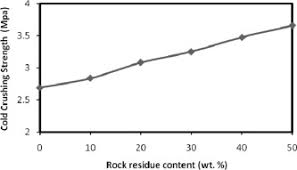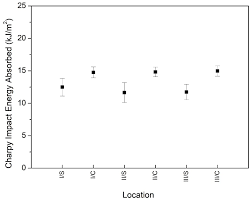## How to Calculate and Solve for Cold Crushing Strength | Refractories

The cold crushing strength is illustrated by the image below.To compute for cold crushing strength, two essential parameters are needed and these parameters are Maximum Load (MI) and Cross-sectional Area (A).

The formula for calculating cold crushing strength:

CCS = MI/A

Where:

CCS = Cold Crushing Strength
A = Cross-sectional Area

Let’s solve an example;
Find the cold crushing strength when the maximum load is 44 and the cross-sectional area is 11.

This implies that;

MI = Maximum Load = 44
A = Cross-sectional Area = 11

CCS = MI/A
CCS = 44/11
CCS = 4

Therefore, the cold crushing strength is 4 Pa.

Calculating the Maximum Load when the Cold Crushing Strength and the Cross-Sectional Area is Given.

MI = CCS x A

Where:

CCS = Cold Crushing Strength
A = Cross-sectional Area

Let’s solve an example;
Given that the cold crushing strength is 15 and the cross-sectional area is 5. Find the maximum load?

This implies that;

CCS = Cold Crushing Strength = 15
A = Cross-sectional Area = 5

MI = CCS x A
MI = 15 x 5
MI = 75

Therefore, the cold crushing strength is 75.

## How to Calculate and Solve for Rod Toughness | Polymer DeformationThe image above represents rod toughness.

To compute for rod toughness, three essential parameters are needed and these parameters are Short Rod Calibration Constant (A), Maximum Load (Pc) and Short Rod Diameter (B).

The formula for calculating rod toughness:

KIC = APcB-3/2

Where:

KIC = Rod Toughness
A = Short Rod Calibration Constant
B = Short Rod Diameter

Let’s solve an example;
Find the rod toughness when the short rod calibration constant is 12, the maximum load is 14 and the short rod diameter is 18.

This implies that;

A = Short Rod Calibration Constant = 12
Pc = Maximum Load = 14
B = Short Rod Diameter = 18

KIC = APcB-3/2
KIC = (12)(14)(18)-3/2
KIC = (12)(14)(0.013)
KIC = 2.199

Therefore, the rod toughness is 2.199 J/m³.